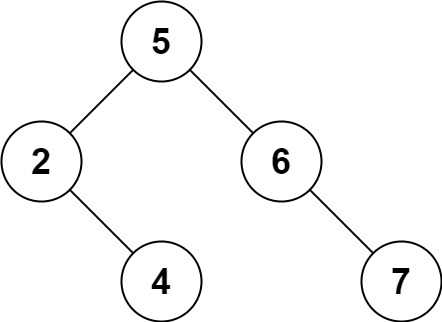Given a root node reference of a BST and a key, delete the node with the given key in the BST. Return the root node reference (possibly updated) of the BST.

Basically, the deletion can be divided into two stages:

1. Search for a node to remove.
2. If the node is found, delete the node.

Follow up: Can you solve it with time complexity `O(height of tree)`?

Example 1:

```Input: root = [5,3,6,2,4,null,7], key = 3
Output: [5,4,6,2,null,null,7]
Explanation: Given key to delete is 3. So we find the node with value 3 and delete it.
One valid answer is [5,4,6,2,null,null,7], shown in the above BST.
Please notice that another valid answer is [5,2,6,null,4,null,7] and it's also accepted.```

Example 2:

```Input: root = [5,3,6,2,4,null,7], key = 0
Output: [5,3,6,2,4,null,7]
Explanation: The tree does not contain a node with value = 0.
```

Example 3:

```Input: root = [], key = 0
Output: []
```

Constraints:

• The number of nodes in the tree is in the range `[0, 104]`.
• `-105 <= Node.val <= 105`
• Each node has a unique value.
• `root` is a valid binary search tree.
• `-105 <= key <= 105`

Solution: (Recursion)

``````/**
* Definition for a binary tree node.
* function TreeNode(val, left, right) {
*     this.val = (val===undefined ? 0 : val)
*     this.left = (left===undefined ? null : left)
*     this.right = (right===undefined ? null : right)
* }
*/
/**
* @param {TreeNode} root
* @param {number} key
* @return {TreeNode}
*/
var deleteNode = function(root, key) {
if (root === null) return root;
if (key > root.val) {
root.right = deleteNode(root.right, key);
} else if (key < root.val) {
root.left = deleteNode(root.left, key);
} else { // found the key
// has children
if (root.left !== null && root.right !== null) {
let min = new TreeNode();
min = root.right;
while (min.left !== null) min = min.left;
root.val = min.val;
root.right = deleteNode(root.right, min.val);
} else { // no children
let newRoot = new TreeNode();
newRoot = root.left === null ? root.right : root.left;
root.left = root.right = null;
return newRoot;
}
}
return root;
};``````